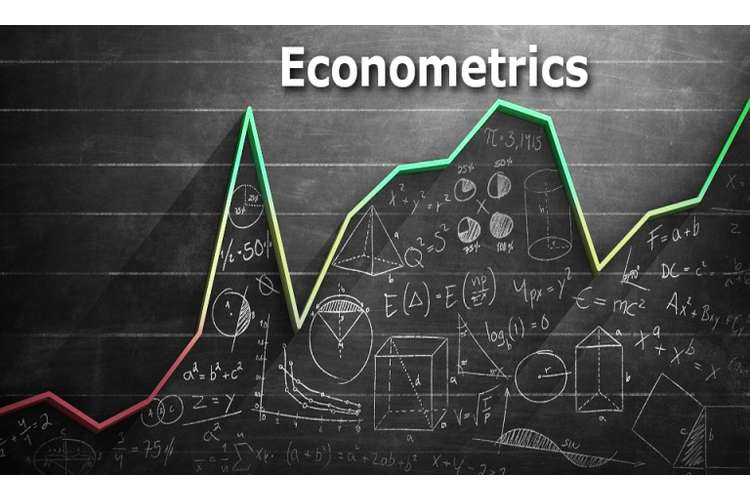# ECON2300 Introduction Of Econometrics Proof Reading Services## Question 3

From; n = 601,

Let, X1be Naffairs – the number of affairs in a given year.

X2 – Age of the respondent

X3– the number of years the respondent has been married

X4 – number of children in the family (Jeffrey, 2009).

X5 – a self-assessed measure of religiosity

X6 – years of education

X7 – a self-assessed measure of marital satisfaction.

Also, let yt – extramarital affairs.

From the model given as;

yt = β0 + β1X1 + β2X2 + β3X3 + μt (Jeffrey, 2009).

yt = β0 + β1number of affairs+ β2age+ β3number of year respondent has been married+ β4number of children in the family+ β5religiosity+ β6years of education7material satisfactiont.

Where yt is the dependent variable (extramarital affairs) while X1, X2,.. X6 are explanatory variables. And μt- error term.β-coefficient.

### Explanation of the model

The model is a good fit since some explanatory variables are significant. This implies that; the number of affairs in a given year significantly predict the extramarital affairs. Age of the respondent is also significant in predicting the model (Roberto, 2013). The number of years the respondent has been married significantly predicts the extramarital affairs. The number of kids in the family also significantly predicts the model. A self-assessed measure of religiosity does not significantly predict the dependent variable. Years of education do not significantly predict the dependent variable. Hence, the more years of healthcare education the lower chances of extramarital affair. A self-assessed measure of marital satisfaction shows an inverse relation with the dependent variable (Roberto, 2013).

The validity of the model varies with the number of explanatory variables used. Many variables used does not necessarily mean a good fit of the model (William, 2012). One of the strengths of the model is that it includes in the number of affairs in the prediction of the extramarital affairs. It is good because it helps policy makers in determining the relationship between the independent variable and dependent (William, 2012).

Similarly, the model is good because it can be used to forecast the results in future. On the other hand, it has weaknesses such as failure to determine to what extent can these explanatory variables explain the dependent variable. It does not clearly give the values that explain the predictor (Dimitros and Stephen. 2011).

Alternatively, the model may depend according to the significance of the explanatory variables. The stronger the independent variables, the higher the predictor. Hence the model becomes;

yt = β0 + β1X1 + β2X2 + β3X3 + μt

yt = β0+ 1.76587LOG–1.6999C – 0.0119TB3+ μt.Similarly, the model is a good fit since p (0.00) <0.05. The model can be used to determine the rate of growth in the nation. Hence more population more demand which increases the production (Dimitros and Stephen. 2011). The specification of this model is appropriate for the data given since n-number of observations were large. In most cases simple models like linear models are preferred since are simple to explain. The standard errors are correct since these tell the deviation in the estimates. It is true because the number of observations was large and gives smaller errors. Multicollinearity does not affect my model since there were no similar independent variables in the same model hence multicollinearity is small (Dimitros and Stephen. 2011).

#### References

1. Asteriou, Dimitros; Hall, Stephen. 2011. "The Classical Linear Regression Model". Applied Econometrics (Second ed.). Palgrave MacMillan. pp. 29–91. ISBN 978-0-230-27182-1.
2. Greene, William . (2012). Econometric Analysis (Sixth ed.). Upper Saddle River: Pearson. ISBN 978-0-13-513740-6.
3. Pedace, Roberto.2013. "Building the Classical Linear Regression Business Model". Econometrics for Dummies. Hoboken, NJ: Wiley. pp. 59–134. ISBN 978-1-118-53384-0.
4. Wooldridge, Jeffrey.(2009). Introductory Econometrics: A Modern Approach (Fourth ed.). Australia: South-Western. p. 88. ISBN 978-0-324-66054-8.